## Portfolio

Bruce Wang's portfolio work - both Drag and General images. A3 Sized Prints - Each are Priced At £90.00.

For different sizes other than A3 please email bruce@citizenwangstudio.com

## Page 48 of 48

• General
• Drag
• Belfast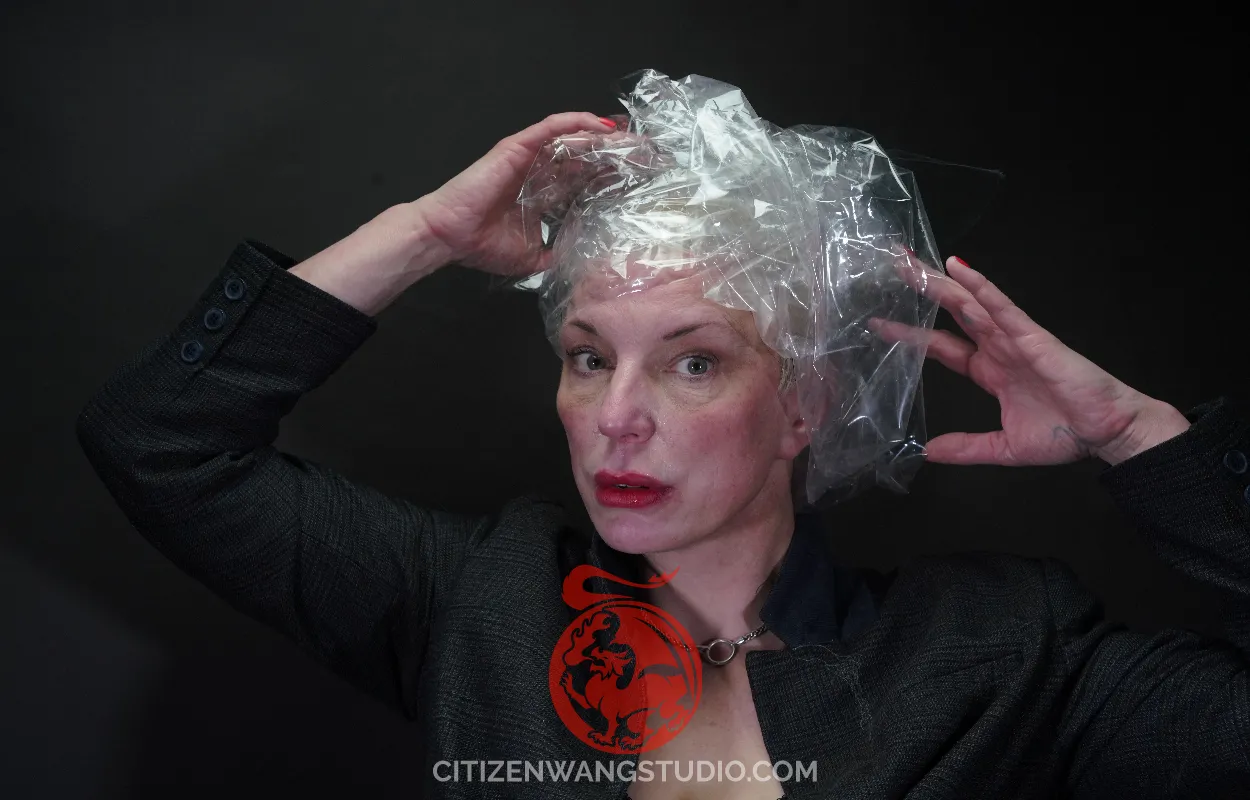Print Number = 12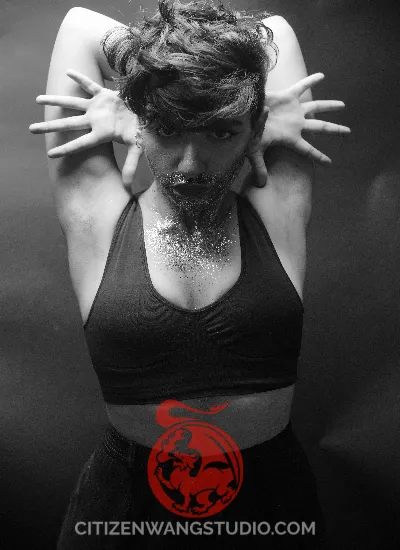Print Number = 11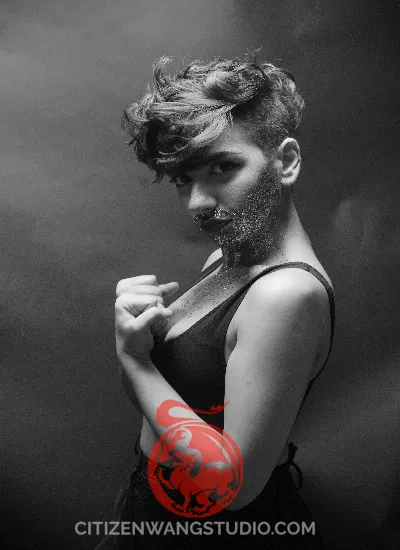Print Number = 10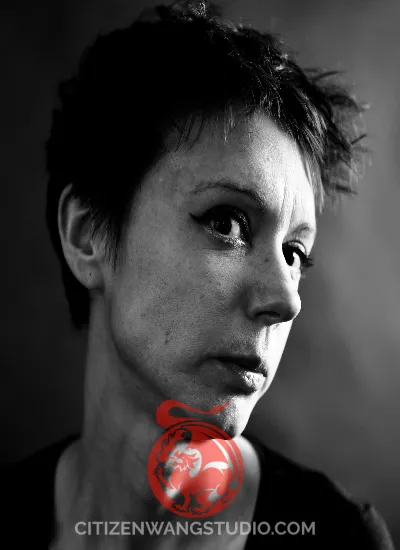Print Number = 9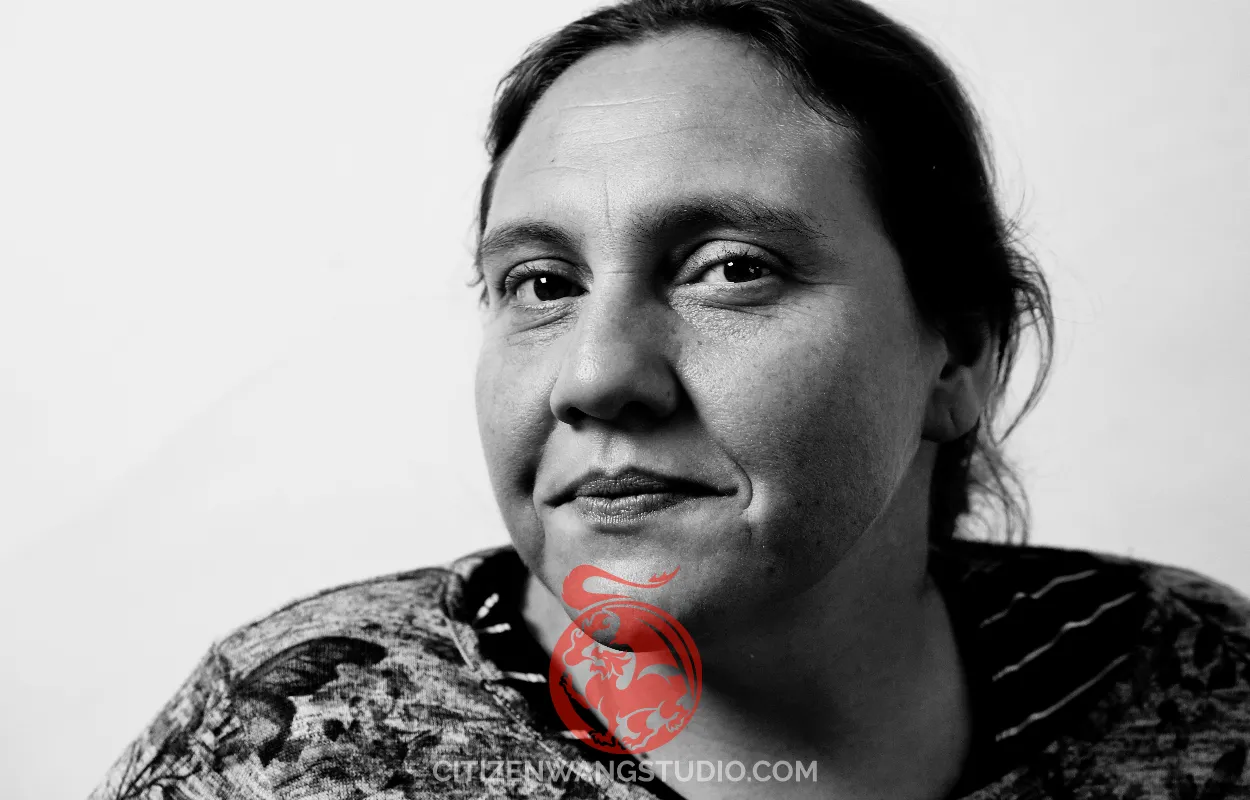Print Number = 8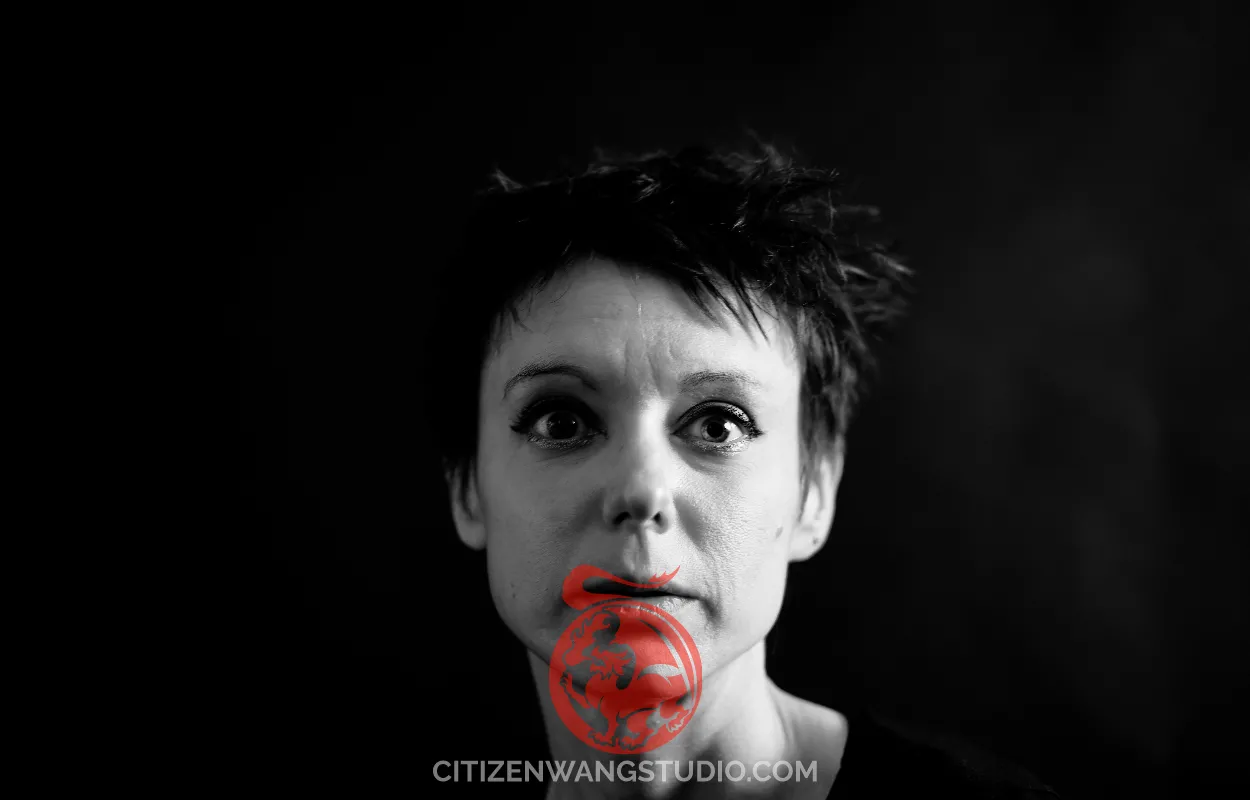Print Number = 7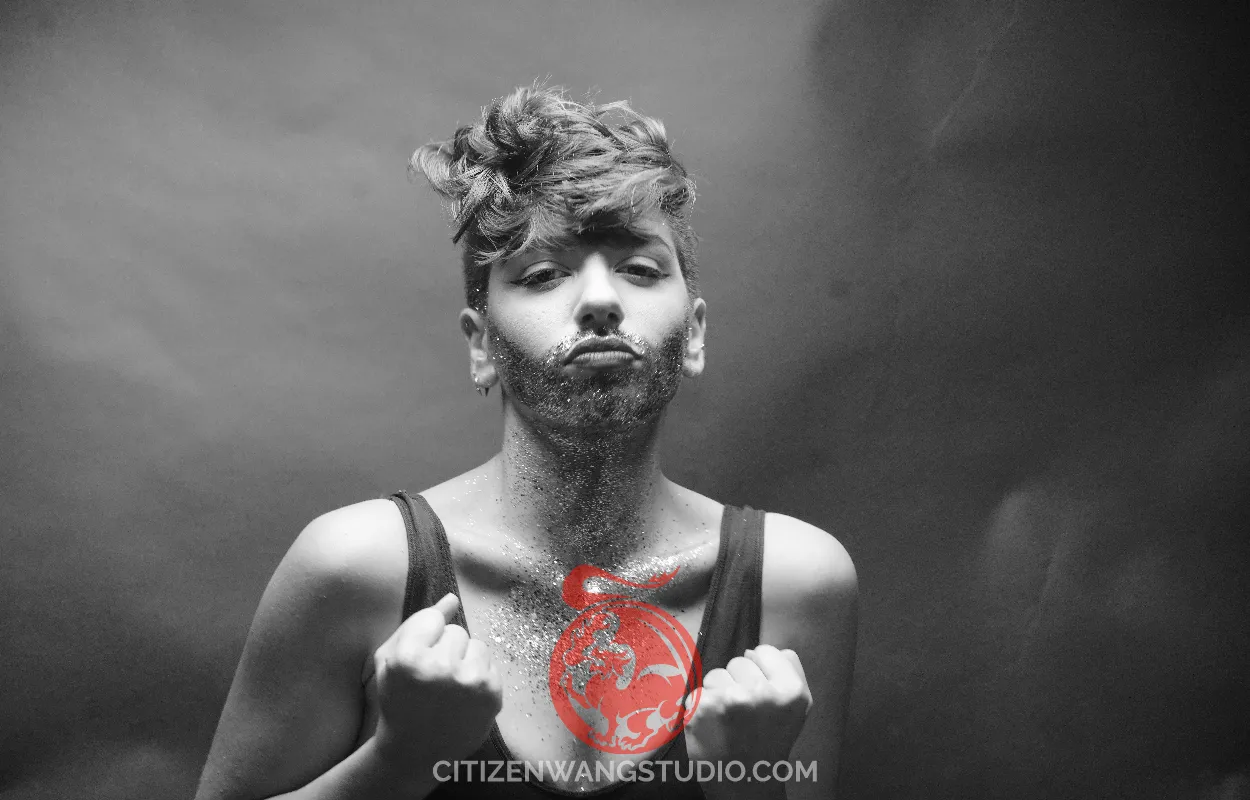Print Number = 6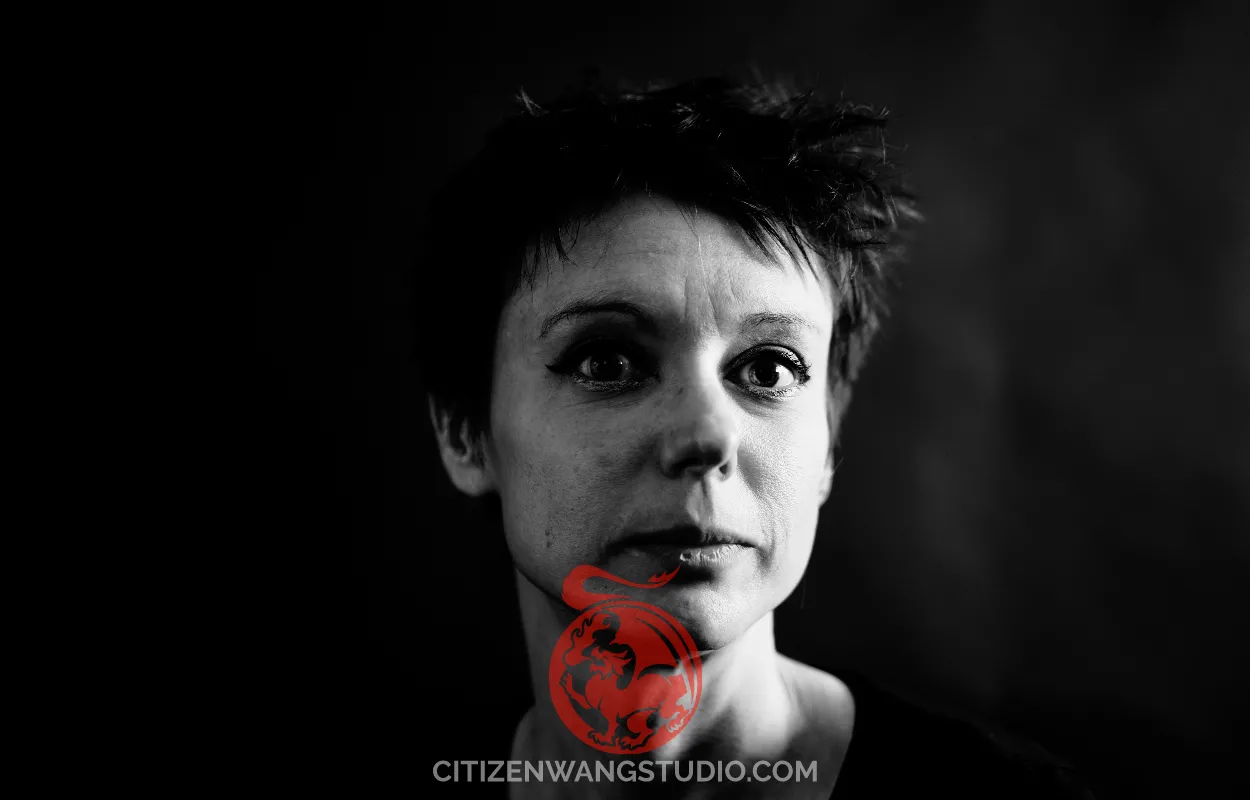Print Number = 5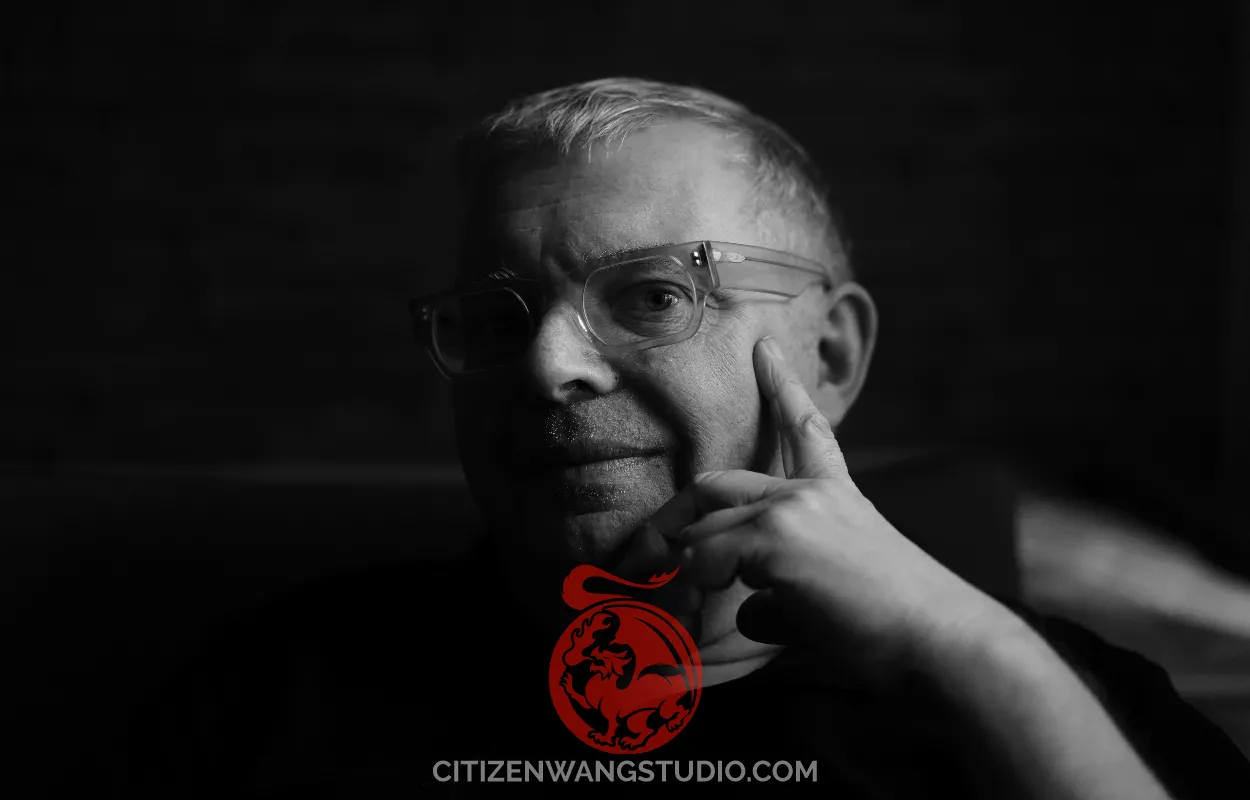Print Number = 4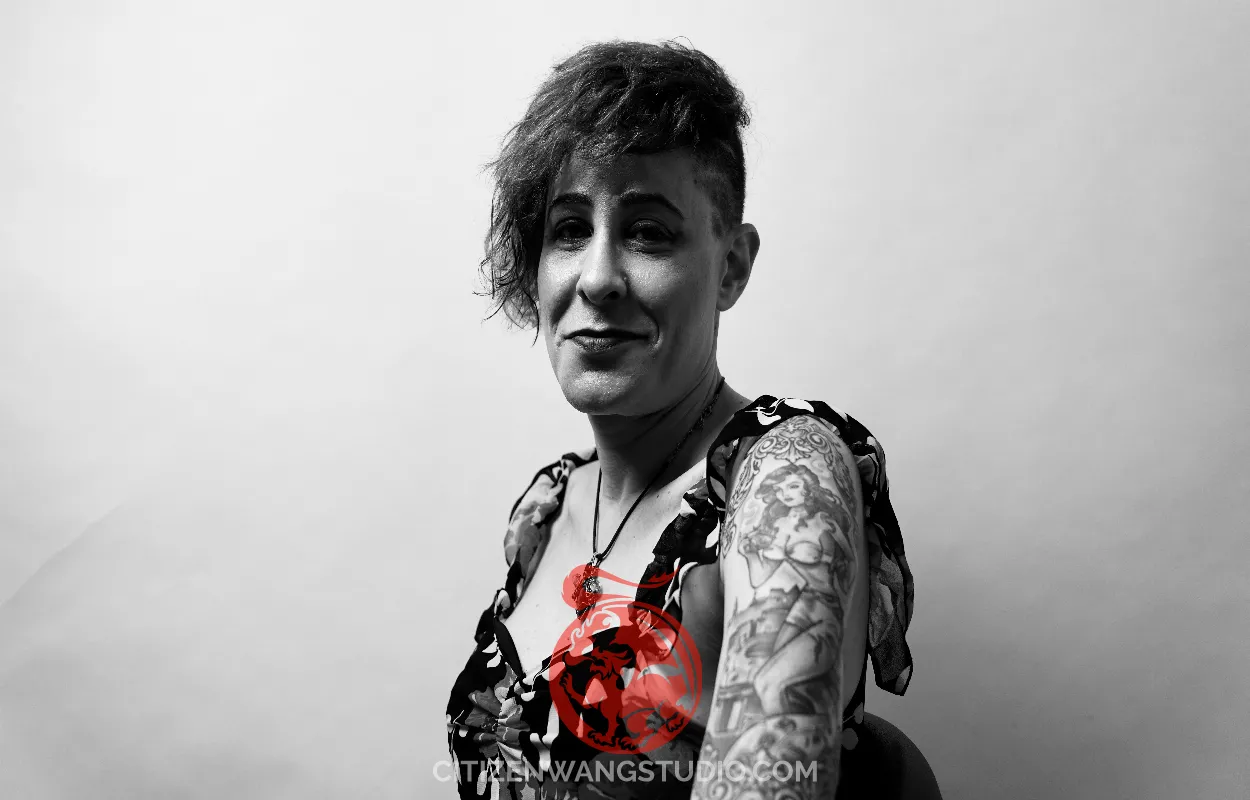Print Number = 3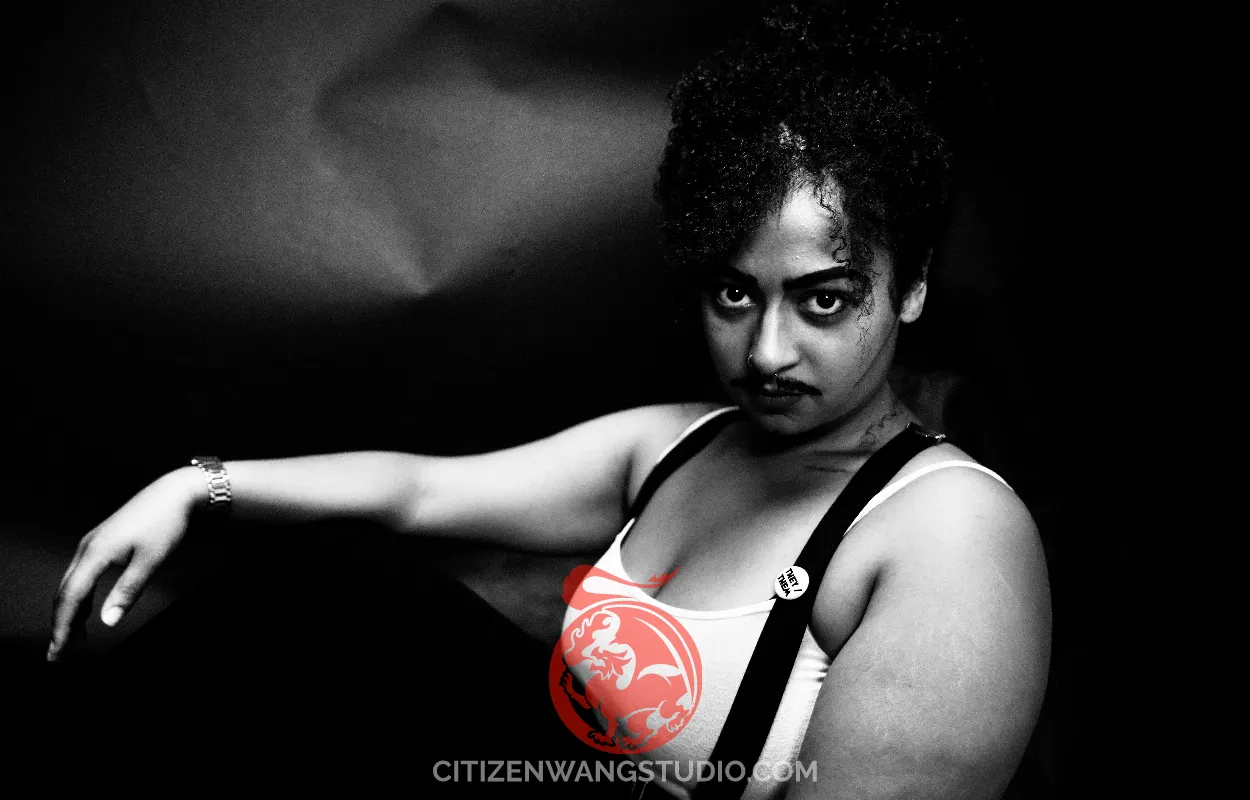Print Number = 2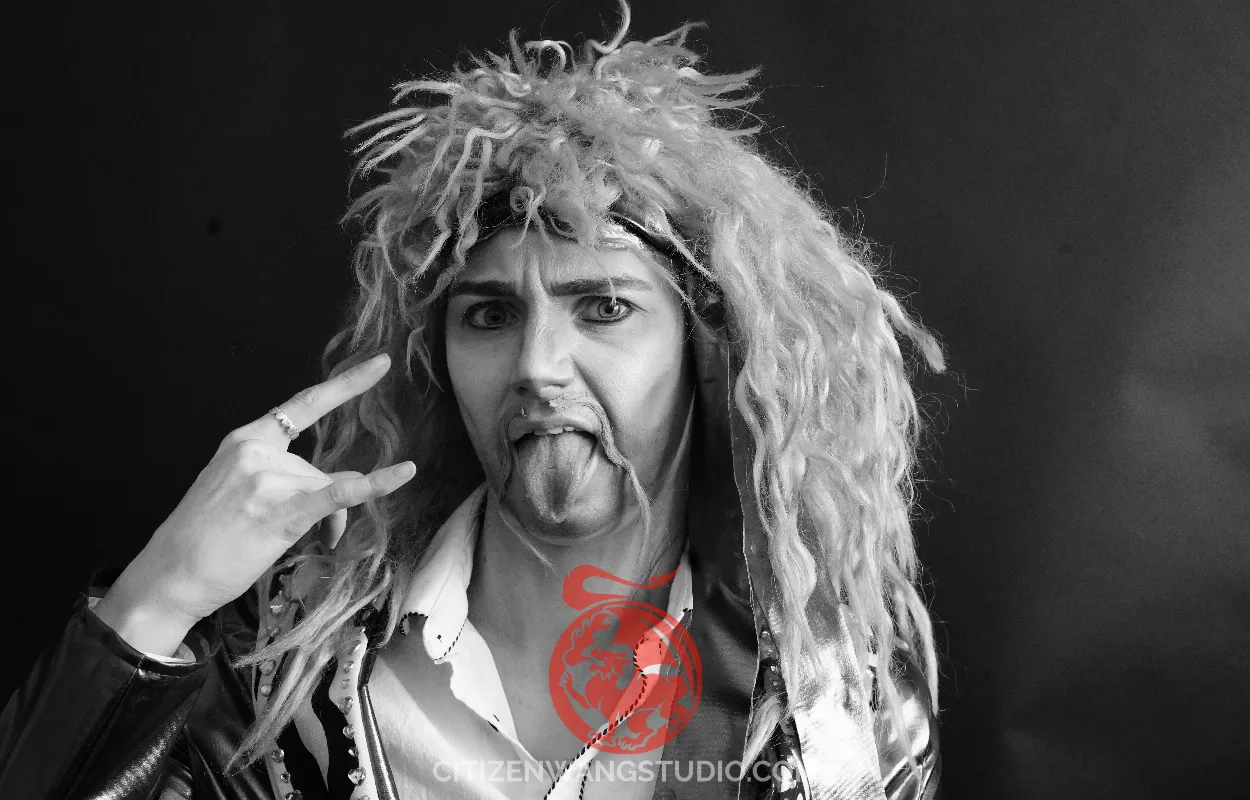Print Number = 1